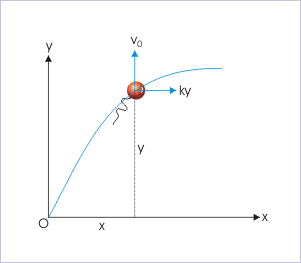# 6.4 Accelerated motion in two dimensions  (Page 5/6)

 Page 5 / 6

## Application

Questions and their answers are presented here in the module text format as if it were an extension of the treatment of the topic. The idea is to provide a verbose explanation, detailing the application of theory. Solution presented is, therefore, treated as the part of the understanding process – not merely a Q/A session. The emphasis is to enforce ideas and concepts, which can not be completely absorbed unless they are put to real time situation.

## Representative problems and their solutions

We discuss problems, which highlight certain aspects of the study leading to the accelerated motion in two dimensions. The questions are categorized in terms of the characterizing features of the subject matter :

• Path of motion
• Tangential and normal accelerations
• Nature of motion
• Displacement in two dimensions

## Path of motion

Problem : A balloon starts rising from the surface with a constant upward velocity, “ ${v}_{0}$ ”. The balloon gains a horizontal drift due to the wind. The horizontal drift velocity is given by “ky”, where “k” is a constant and “y” is the vertical height of the balloon from the surface. Derive an expression of path of the motion.

Solution : An inspection of the equation of drift velocity (v = ky) suggests that balloon drifts more with the gain in height. A suggestive x-y plot of the motion is shown here.The balloon moves with an acceleration in horizontal direction.

Let vertical and horizontal direction corresponds to “y” and “x” axes of the coordinate system. Here,

${v}_{y}={v}_{0}$

${v}_{x}={k}_{y}$

We are required to know the relation between vertical and horizontal components of displacement from the expression of component velocities. It means that we need to know a lower order attribute from higher order attribute. Thus, we shall proceed with integration of differential equation, which defines velocity as :

$⇒\frac{đx}{đt}=ky$

Similarly,

$\frac{đy}{đt}={v}_{0}$

$⇒đy={v}_{0}đt$

Combining two equations by eliminating “dt”,

$dx=\frac{kyđy}{{v}_{0}}$

Now, integrating both sides, we have :

$x=\int \frac{kyđy}{{v}_{0}}$

Taking out constants out of the integral,

$⇒x=\frac{k}{{v}_{0}}\int yđy$

$x=\frac{k{y}^{2}}{2{v}_{0}}$

This is the required equation of motion, which is an equation of a parabola. Thus, the suggested plot given in the beginning, as a matter of fact, was correct.

## Tangential and normal accelerations

Problem : A balloon starts rising from the surface with a constant upward velocity, “ ${v}_{0}$ ”. The balloon gains a horizontal drift due to the wind. The horizontal drift velocity is given by “ky”, where “k” is a constant and “y” is the vertical height of the balloon from the surface. Derive expressions for the tangential and normal accelerations of the balloon.

Solution : We can proceed to find the magnitude of total acceleration by first finding the expression of velocity. Here, velocity is given as :

$v=kyi+{v}_{0}j$

Since acceleration is higher order attribute, we obtain its expression by differentiating the expression of velocity with respect to time :

$⇒a=\frac{đv}{đt}=k{v}_{y}i=k{v}_{0}i$

It is obvious that acceleration is one dimensional. It is evident from the data given also. The balloon moving with constant vertical velocity has no acceleration in y-direction. The speed of the balloon in x-direction, however, keeps changing with height (time) and as such total acceleration of the balloon is in x-direction. The magnitude of total acceleration is :

#### Questions & Answers

What are the system of units
Jonah Reply
A stone propelled from a catapult with a speed of 50ms-1 attains a height of 100m. Calculate the time of flight, calculate the angle of projection, calculate the range attained
Samson Reply
58asagravitasnal firce
Amar
water boil at 100 and why
isaac Reply
what is upper limit of speed
Riya Reply
what temperature is 0 k
Riya
0k is the lower limit of the themordynamic scale which is equalt to -273 In celcius scale
Mustapha
How MKS system is the subset of SI system?
Clash Reply
which colour has the shortest wavelength in the white light spectrum
Mustapha Reply
how do we add
Jennifer Reply
if x=a-b, a=5.8cm b=3.22 cm find percentage error in x
Abhyanshu Reply
x=5.8-3.22 x=2.58
sajjad
what is the definition of resolution of forces
Atinuke Reply
what is energy?
James Reply
Ability of doing work is called energy energy neither be create nor destryoed but change in one form to an other form
Abdul
motion
Mustapha
highlights of atomic physics
Benjamin
can anyone tell who founded equations of motion !?
Ztechy Reply
n=a+b/T² find the linear express
Donsmart Reply
أوك
عباس
Quiklyyy
Sultan Reply
Moment of inertia of a bar in terms of perpendicular axis theorem
Sultan Reply
How should i know when to add/subtract the velocities and when to use the Pythagoras theorem?
Yara Reply

### Read also:

#### Get the best Physics for k-12 course in your pocket!

Source:  OpenStax, Physics for k-12. OpenStax CNX. Sep 07, 2009 Download for free at http://cnx.org/content/col10322/1.175
Google Play and the Google Play logo are trademarks of Google Inc.

Notification Switch

Would you like to follow the 'Physics for k-12' conversation and receive update notifications?ByBy Nick SwainBy Mariah HauptmanBy Gerr Zen